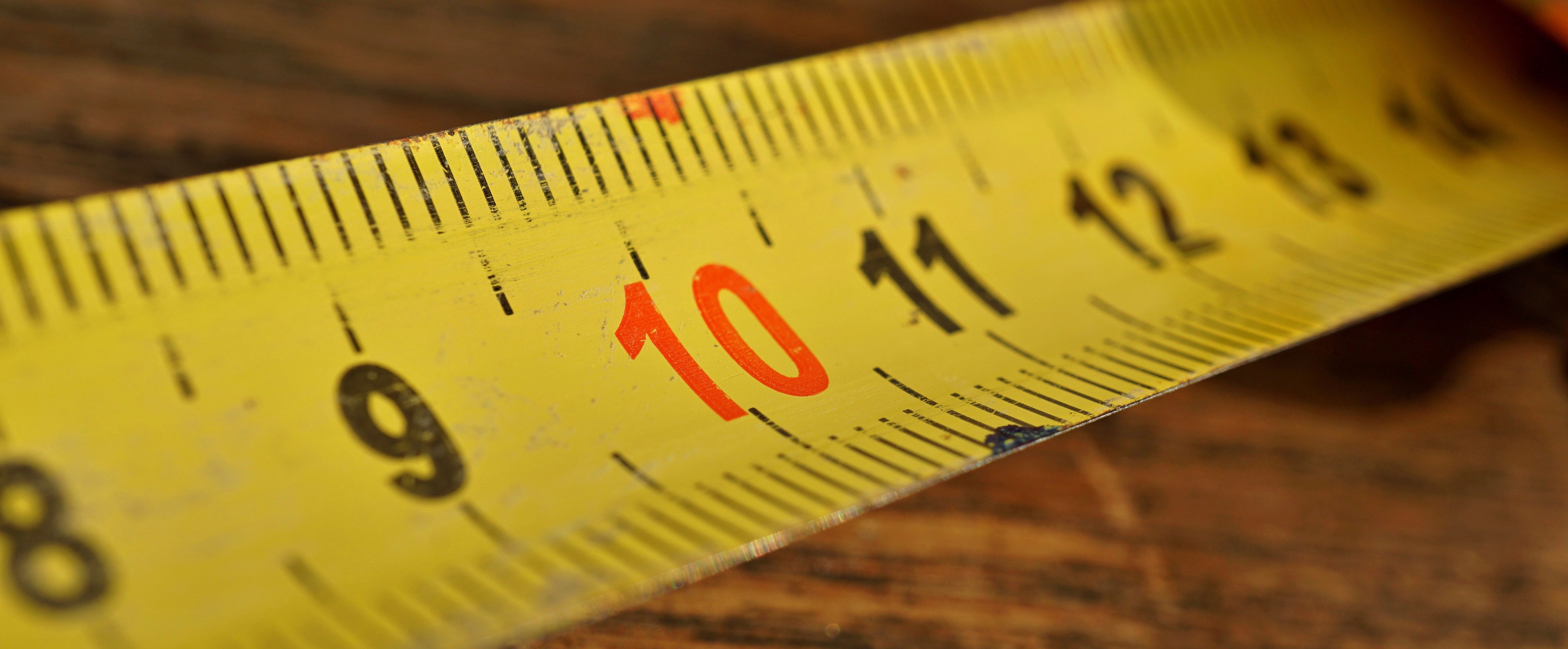## Translate

### What is Length (L) ? Conversions and Formulas In PDF FileIn geometric measurements, length is the most extended dimension of an object. In the International System of Quantities, length is any quantity with dimension distance. In other contexts "length" is the measured dimension of an object. For example, it is possible to cut a length of a wire which is shorter than wire thickness.

Length may be distinguished from height, which is vertical extent, and width or breadth, which are the distance from side to side, measuring across the object at right angles to the length. Length is a measure of one dimension, whereas area is a measure of two dimensions (length squared) and volume is a measure of three dimensions (length cubed). In most systems of measurement, the unit of length is a base unit, from which other units are derived.

Conversions and Formulas

Length Units

 Millimetres Centimetres Meters Kilometres Inches Feet Yards Miles mm cm m km in ft yd mi 1 0.1 0.001 0.000001 0.03937 0.003281 0.001094 6.21e-07 10 1 0.01 0.00001 0.393701 0.032808 0.010936 0.000006 1000 100 1 0.001 39.37008 3.28084 1.093613 0.000621 1000000 100000 1000 1 39370.08 3280.84 1093.613 0.621371 25.4 2.54 0.0254 0.000025 1 0.083333 0.027778 0.000016 304.8 30.48 0.3048 0.000305 12 1 0.333333 0.000189 914.4 91.44 0.9144 0.000914 36 3 1 0.000568 1609344 160934.4 1609.344 1.609344 63360 5280 1760 1

Surveyor’s Measure

 7.92 Inches = 1 Link 25 Links = 1 Rod 4 Rods = 1 Chain = 66 Feet 10 Square Chains = 1 Acre 160 Square Rods = 1 Acre 640 Acres = 1 Square Mile 36 Square Miles (6 miles sq.) = 1 Township

Formulas For length

 Inches x 0.0833 = Feet Inches x 0.028 = Yards Feet x 12 = Inches Feet x 0.33 = Yards Feet x 0.06 = Rods Feet x 1.5 = Links Yards x 36 = Inches Yards x 3 = Feet Yards x 0.18 = Rods Links x .22 = Yards Links x .66 = Feet Rods x 198 = Inches Rods x 16.5 = Feet Rods x 5.5 = Yards Miles x 5,280 = Feet Miles x 1,760 = Yards Miles x 320 = Rods

 Inches x 25.40 = Millimetres (mm) Inches x 2.540 = Centimetres (cm) Inches x 0.0254 = Meters (m) Feet x 0.305 = Meters (m) Yards x 0.91 = Meters (m) Rods x 0.199 = Meters (m) Miles x 1.61 = Kilometres (km)

Download PDF Files